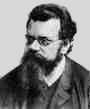Home

Lecture Notes

PHY 418: Statistical Mechanics I
Prof. S. Teitel stte@pas.rochester.edu ---- Spring 2007

## Lecture Notes

The lecture note files correspond roughly to the material presented in a given day's lecture. But you may on occassion find the end of one day's lecture at the start of the file for the next day's lecture, so please look there if you think there might be something missing.

• Lecture 0 - Sketching the history of statistical mechanics and thermodynamics (another such page here.)

• Lecture 1 - Postulates of classical thermodynamics, entropy, temperature, pressure, chemical potential

• Lecture 2 - Conditions for thermal. mechanical and chemical equilibrium, concavity of the entropy, the Euler relation, equations of state, the Gibb-Duhem relation, entropy of the ideal gas

• Lecture 3 - Energy minimum principle, Legendre transformations, Helmholtz free energy

• Lecture 4 - Enthalpy, Gibbs free energy, Grand Potential, heat reservior, extrema principles for thermodynamic potentials, Maxwell's relations

• Lecture 5 - Response functions (specific heat, compressibility, thermal expansion) and relations between them, stability and curvature of free energies

• Something Extra! - Heat engines and the Carnot cycle

• Lecture 6 - Kinetic theory of the ideal gas, Maxwell's velocity distribution, statistical ensembles and the ergodic hypothesis

• Lecture 7 - Liouville's theorem, microcanonical ensemble, density of states and number of states, connection to entropy

• Lecture 8 - Entropy of the ideal gas, entropy of mixing and Gibbs paradox, indistinguishable particles, Sackur-Tetrode equation

• Lecture 9 - Canonical ensemble, energy fluctuations and specific heat, equivalence of microcanonical and canonical ensembles

• Lecture 10 - Average energy vs most probably energy, proof of Stirling's formula, factorization of canonical partition function for non-interactng particles, ideal gas

• Lecture 11 - Virial and equipartition theorems, elastic vibrations of solids and the Law of Dulong and Petit, paramagnetism and the Curie Law (note: the 3rd from last page of these notes is out of order - it should be the last page!)

• Lecture 12 - Entropy and information theory

• Lecture 13 - The grand canonical ensemble, fluctuations of energy and number of particles

• Lecture 14 - The grand canonical partition function for non-interacting degrees of freedom, chemical equilibrium, adsorption sites

• Lecture 15 - The density operator and quantum ensembles

• Lecture 16 - Quantum many particle systems, symmetry of the wavefunction, boson and fermions, non-interacting particles

• Lecture 17 - Two particle density matrix in real space, N particle partition function in real space representation, grand canonical partition function for non-interacting fermions and bosons

• Lecture 18 - Average occupation numbers, comparison with and validity of the classical limit of a quantum ideal gas, the harmonic oscillator and bosons

• Lecture 19 - Debye model for the specific heat due to ionic vibrations, black body radiation

• Lecture 20 - Ideal quantum gas of fermions or bosons, density, pressure, energy, classical limit

• Lecture 21 - Degenerate fermi gas, Sommerfeld model of electrons in a metal, Fermi energy, specific heat

• Lecture 22 - Pauli paramagnetism of an ideal fermi gas

• Lecture 23 - Ideal gas of bosons, Bose-Einstein condensation

• Lecture 24 - Bose-Einstein condensation in laser cooled atomic gases, classical spin models and ensembles

• Lecture 25 - Ising model, phase transitions and the thermodynamic limit, phase diagram

• Lecture 26 - Mean field approximation for the Ising model, graphical solution

• Lecture 27 - Critical exponents, Maxwell construction, Landau's theory of phase transitions

• Lecture 28 - Critical exponents in Landau theory, exact solution of 1D Ising model

• Lecture 29 - Landau-Ginzburg theory, correlation length, fluctuations and the upper critical dimension